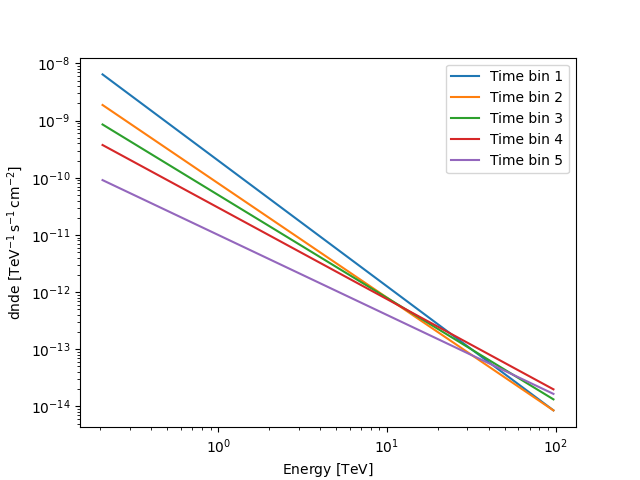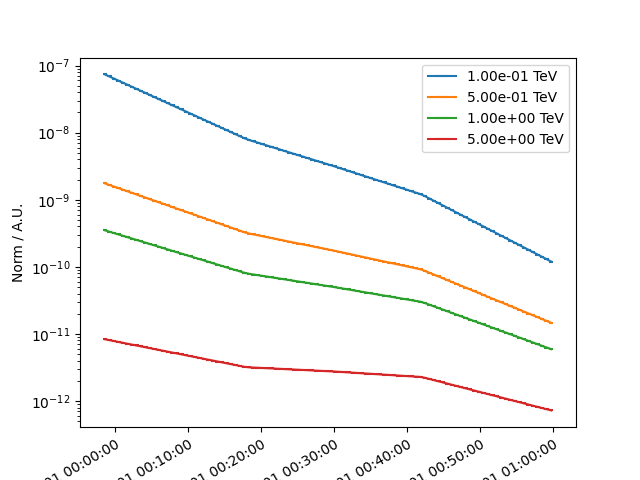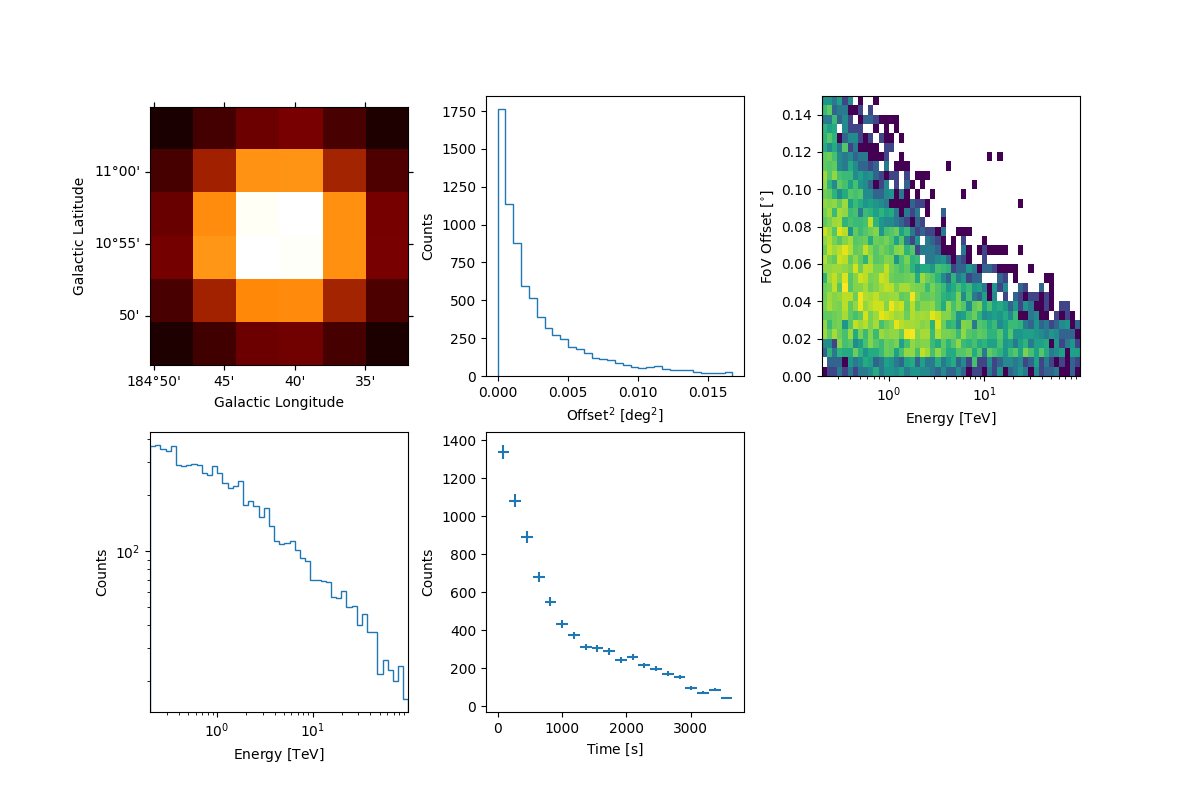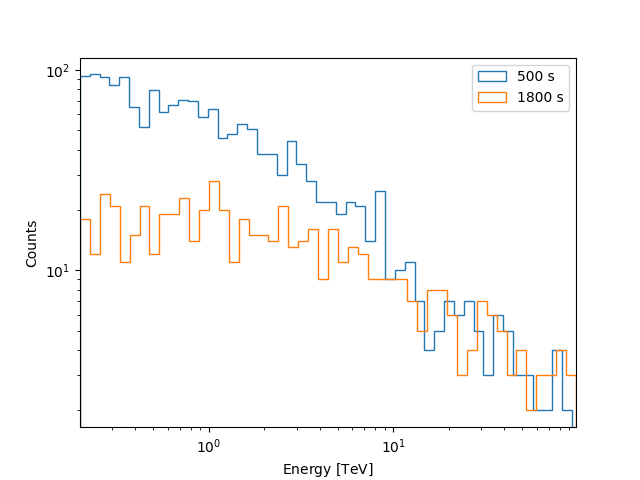# Sample a source with energy-dependent temporal evolution#

This notebook shows how to sample events of sources whose model evolves in energy and time.

## Prerequisites#

To understand how to generate a model and a MapDataset and how to fit the data, please refer to the `SkyModel` and 3D map simulation tutorial. To know how to sample events for standards sources, we suggest to visit the event sampler Event sampling tutorial.

## Objective#

Describe the process of sampling events of a source having an energy-dependent temporal model, and obtain an output event-list.

## Proposed approach#

Here we will show how to create an energy dependent temporal model; then we also create an observation and define a Dataset object. Finally, we describe how to sample events from the given model.

We will work with the following functions and classes:

## Setup#

```from pathlib import Path
import astropy.units as u
from astropy.coordinates import Angle, SkyCoord
from astropy.time import Time
from regions import CircleSkyRegion, PointSkyRegion
import matplotlib.pyplot as plt
from gammapy.data import Observation, observatory_locations
from gammapy.datasets import MapDataset, MapDatasetEventSampler
from gammapy.makers import MapDatasetMaker
from gammapy.maps import MapAxis, RegionNDMap, WcsGeom
from gammapy.modeling.models import (
ConstantSpectralModel,
FoVBackgroundModel,
LightCurveTemplateTemporalModel,
PointSpatialModel,
PowerLawSpectralModel,
SkyModel,
)
```

## Check setup#

```from gammapy.utils.check import check_tutorials_setup

check_tutorials_setup()
```
```System:

python_executable      : /home/runner/work/gammapy-docs/gammapy-docs/gammapy/.tox/build_docs/bin/python
python_version         : 3.9.16
machine                : x86_64
system                 : Linux

Gammapy package:

version                : 1.1
path                   : /home/runner/work/gammapy-docs/gammapy-docs/gammapy/.tox/build_docs/lib/python3.9/site-packages/gammapy

Other packages:

numpy                  : 1.24.3
scipy                  : 1.10.1
astropy                : 5.2.2
regions                : 0.7
click                  : 8.1.3
yaml                   : 6.0
IPython                : 8.14.0
jupyterlab             : not installed
matplotlib             : 3.7.1
pandas                 : not installed
healpy                 : 1.16.2
iminuit                : 2.21.3
sherpa                 : 4.15.1
naima                  : 0.10.0
emcee                  : 3.1.4
corner                 : 2.2.2
ray                    : 2.5.0

Gammapy environment variables:

GAMMAPY_DATA           : /home/runner/work/gammapy-docs/gammapy-docs/gammapy-datasets/1.1
```

## Create the energy-dependent temporal model#

The source we want to simulate has a spectrum that varies as a function of the time. Here we show how to create an energy dependent temporal model. If you already have such a model, go directly to the corresponding section.

In the following example, the source spectrum will vary continuously with time. Here we define 5 time bins and represent the spectrum at the center of each bin as a powerlaw. The spectral evolution is also shown in the following plot:

```amplitudes = u.Quantity(
[2e-10, 8e-11, 5e-11, 3e-11, 1e-11], unit="cm-2s-1TeV-1"
)  # amplitude
indices = u.Quantity([2.2, 2.0, 1.8, 1.6, 1.4], unit="")  # index

for i in range(len(amplitudes)):
spec = PowerLawSpectralModel(
index=indices[i], amplitude=amplitudes[i], reference="1 TeV"
)
spec.plot([0.2, 100] * u.TeV, label=f"Time bin {i+1}")
plt.legend()
plt.show()
```Let’s now create the temporal model (if you already have this model, please go directly to the `Read the energy-dependent model` section), that will be defined as a `LightCurveTemplateTemporalModel`. The latter take as input a `RegionNDMap` with temporal and energy axes, on which the fluxes are stored.

To create such map, we first need to define a time axis with `MapAxis`: here we consider 5 time bins of 720 s (i.e. 1 hr in total). As a second step, we create an energy axis with 10 bins where the powerlaw spectral models will be evaluated.

```# source position
position = SkyCoord("100 deg", "30 deg", frame="icrs")

# time axis
time_axis = MapAxis.from_bounds(0 * u.s, 3600 * u.s, nbin=5, name="time", interp="lin")

# energy axis
energy_axis = MapAxis.from_energy_bounds(
energy_min=0.2 * u.TeV, energy_max=100 * u.TeV, nbin=10
)
```

Now let’s create the `RegionNDMap` and fill it with the expected spectral values:

```# create the RegionNDMap containing fluxes
m = RegionNDMap.create(
region=PointSkyRegion(center=position),
axes=[energy_axis, time_axis],
unit="cm-2s-1TeV-1",
)

# to compute the spectra as a function of time we extract the coordinates of the geometry
coords = m.geom.get_coord()

# We reshape the indices and amplitudes array to perform broadcasting
indices = indices.reshape(coords["time"].shape)
amplitudes = amplitudes.reshape(coords["time"].shape)

# evaluate the spectra and fill the RegionNDMap
m.quantity = PowerLawSpectralModel.evaluate(
coords["energy"], indices, amplitudes, 1 * u.TeV
)
```

### Create the temporal model and write it to disk#

Now, we define the `LightCurveTemplateTemporalModel`. It needs the map we created above and a reference time. The latter is crucial to evaluate the model as a function of time. We show also how to write the model on disk, noting that we explicitly set the `format` to `map`.

```t_ref = Time(51544.00074287037, format="mjd", scale="tt")
filename = "./temporal_model_map.fits"
temp = LightCurveTemplateTemporalModel(m, t_ref=t_ref, filename=filename)
temp.write(filename, format="map", overwrite=True)
```

We read the map written on disc again with `LightCurveTemplateTemporalModel.read`. When the model is from a map, the arguments `format="map"` is mandatory. The map is `fits` file, with 3 extensions:

1) `SKYMAP`: a table with a `CHANNEL` and `DATA` column; the number of rows is given by the product of the energy and time bins. The `DATA` represent the values of the model at each energy;

2) `SKYMAP_BANDS`: a table with `CHANNEL`, `ENERGY`, `E_MIN`, `E_MAX`, `TIME`, `TIME_MIN` and `TIME_MAX`. `ENERGY` is the mean of `E_MIN` and `E_MAX`, as well as `TIME` is the mean of `TIME_MIN` and `TIME_MAX`; this extension should contain the reference time in the header, through the keywords `MJDREFI` and `MJDREFF`.

3) `SKYMAP_REGION`: it gives information on the spatial morphology, i.e. `SHAPE` (only `point` is accepted), `X` and `Y` (source position), `R` (the radius if extended; not used in our case) and `ROTANG` (the angular rotation of the spatial model, if extended; not used in our case).

```temporal_model = LightCurveTemplateTemporalModel.read(filename, format="map")
```

We note that an interpolation scheme is also provided when loading a map: for an energy-dependent temporal model, the `method` and `values_scale` arguments by default are set to `linear` and `log`. We warn the reader to carefully check the interpolation method used for the time axis while creating the template model, as different methods provide different results. By default, we assume `linear` interpolation for the time, `log` for the energies and values. Users can modify the `method` and `values_scale` arguments but we warn that this should be done only when the user knows the consequences of the changes. Here, we show how to set them explicitly:

```temporal_model.method = "linear"  # default
temporal_model.values_scale = "log"  # default
```

We can have a visual inspection of the temporal model at different energies:

```time_range = temporal_model.reference_time + [-100, 3600] * u.s

temporal_model.plot(time_range=time_range, energy=[0.1, 0.5, 1, 5] * u.TeV)
plt.semilogy()
plt.show()
```## Prepare and run the event sampler#

### Define the simulation source model#

Now that the temporal model is complete, we create the whole source `SkyModel`. We define its spatial morphology as `point-like`. This is a mandatory condition to simulate energy-dependent temporal model. Other morphologies will raise an error! Note also that the source `spectral_model` is a `ConstantSpectralModel`: this is necessary and mandatory, as the real source spectrum is actually passed through the map.

```spatial_model = PointSpatialModel.from_position(position)
spectral_model = ConstantSpectralModel(const="1 cm-2 s-1 TeV-1")

model = SkyModel(
spatial_model=spatial_model,
spectral_model=spectral_model,
temporal_model=temporal_model,
name="test-source",
)

bkg_model = FoVBackgroundModel(dataset_name="my-dataset")

models = [model, bkg_model]
```

### Define an observation and make a dataset#

In the following, we define an observation of 1 hr with CTA in the alpha-configuration for the south array, and we also create a dataset to be passed to the event sampler. The full `SkyModel` created above is passed to the dataset.

```path = Path("\$GAMMAPY_DATA/cta-caldb")
irf_filename = "Prod5-South-20deg-AverageAz-14MSTs37SSTs.180000s-v0.1.fits.gz"

pointing = SkyCoord(100.0, 30.0, frame="icrs", unit="deg")
livetime = 1 * u.hr

location = observatory_locations["cta_south"]

observation = Observation.create(
obs_id=1001,
pointing=pointing,
livetime=livetime,
irfs=irfs,
location=location,
)
```
```/home/runner/work/gammapy-docs/gammapy-docs/gammapy/.tox/build_docs/lib/python3.9/site-packages/gammapy/data/observations.py:226: GammapyDeprecationWarning: Pointing will be required to be provided as FixedPointingInfo
warnings.warn(
```
```energy_axis = MapAxis.from_energy_bounds("0.2 TeV", "100 TeV", nbin=5, per_decade=True)
energy_axis_true = MapAxis.from_energy_bounds(
"0.05 TeV", "150 TeV", nbin=10, per_decade=True, name="energy_true"
)
migra_axis = MapAxis.from_bounds(0.5, 2, nbin=150, node_type="edges", name="migra")

geom = WcsGeom.create(
skydir=pointing,
width=(2, 2),
binsz=0.02,
frame="icrs",
axes=[energy_axis],
)
```
```empty = MapDataset.create(
geom,
energy_axis_true=energy_axis_true,
migra_axis=migra_axis,
name="my-dataset",
)
maker = MapDatasetMaker(selection=["exposure", "background", "psf", "edisp"])
dataset = maker.run(empty, observation)

dataset.models = models

print(dataset.models)
```
```DatasetModels

Component 0: SkyModel

Name                      : test-source
Datasets names            : None
Spectral model type       : ConstantSpectralModel
Spatial  model type       : PointSpatialModel
Temporal model type       : LightCurveTemplateTemporalModel
Parameters:
const                         :      1.000   +/-    0.00 1 / (cm2 s TeV)
lon_0                         :    100.000   +/-    0.00 deg
lat_0                         :     30.000   +/-    0.00 deg
t_ref                 (frozen):  51544.000       d

Component 1: FoVBackgroundModel

Name                      : my-dataset-bkg
Datasets names            : ['my-dataset']
Spectral model type       : PowerLawNormSpectralModel
Parameters:
norm                          :      1.000   +/-    0.00
tilt                  (frozen):      0.000
reference             (frozen):      1.000       TeV
```

### Let’s simulate the model#

Initialize and run the `MapDatasetEventSampler` class. We also define the `oversample_energy_factor` arguments: this should be carefully considered by the user, as a higher `oversample_energy_factor` gives a more precise source flux estimate, at the expense of computational time. Here we adopt an `oversample_energy_factor` of 10:

```sampler = MapDatasetEventSampler(random_state=0, oversample_energy_factor=10)
events = sampler.run(dataset, observation)
```

Let’s inspect the simulated events in the source region:

```src_position = SkyCoord(100.0, 30.0, frame="icrs", unit="deg")

src_events = events.select_region(on_region)

src_events.peek()
plt.show()
```Let’s inspect the simulated events as a function of time:

```time_interval = temporal_model.reference_time + [300, 700] * u.s
src_events.select_time(time_interval).plot_energy(label="500 s")

time_interval = temporal_model.reference_time + [1600, 2000] * u.s
src_events.select_time(time_interval).plot_energy(label="1800 s")

plt.legend()
plt.show()
```## Exercises#

• Try to create a temporal model with a more complex energy-dependent evolution;